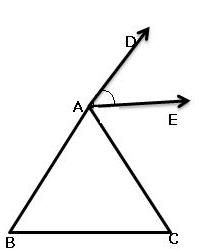# In the given figure, AE bisects Angle DAC and Angle B= Angle C, prove that AE is parallel to BCDAC =B +C (EXTERIOR ANGLE = SUM OF INTERIOR OPPOSITE ANGLES)

DAC = 2C AS (B =C) GIVEN

1/2DAC =C (DEVIDING BY 2)

EAC = C (1/2DAC =EAC) GIVEN

HENCE AE IS PARALLEL TO BC AS ALTERNATE INTERIOR ANGLES ARE EQUAL

• 32
What are you looking for?